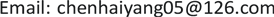1. 引言

m v = ( m + d m ) ( v + d v ) + ( − d m ) ( v − u ) (1)

m v = ( m + d m ) ( v + d v ) + ( − d m ) ( v + d v − u ) (2)

2. 变质量运动火箭运动方程的推导

( m − d m ) d v + C d m = 0 (3)

(3)式展开后可得：

m d v − d m d v + C d m = 0 (4)

m d v + C d m = 0 (5)

− d v C = d m m (6)

v = C ln M 0 M (8)

3. 结论